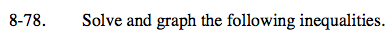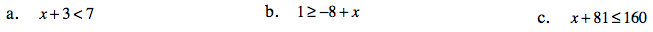Home > CC1 > Chapter 8 > Lesson 8.2.1 > Problem8-78

8-78.
1. Solve and graph the following inequalities: Homework Help ✎

1. x + 3 < 7

2. 1 ≥ −8 + x

3. x + 81 ≤ 160Solve the inequality as you would solve any equation.
(For part (a), subtract 3 from both sides to find the value of x.)

Change the problem to:
x + 3 = 7
x + 3 − 3 = 7 − 3
x = 4

Plot the value of x on a number line.
Open circle (does not include the number itself): > or <
Closed circle (includes the number): ≥ or ≤

Substitute one number from each side of the point you just plotted.
Decide whether each answer is true or false. Point the arrow in the direction of the true value.

Substitute a number less than 4 in for x (let's try 1):
(1) + 3 < 7
4 < 7
TRUE!

Now substitute a number greater than 4 in for x (let's try 5):
(5) + 3 < 7
8 < 7
FALSE!
So the arrow must point to the left (towards the numbers less than 4) because
those numbers give a true answer when substituted in for x.